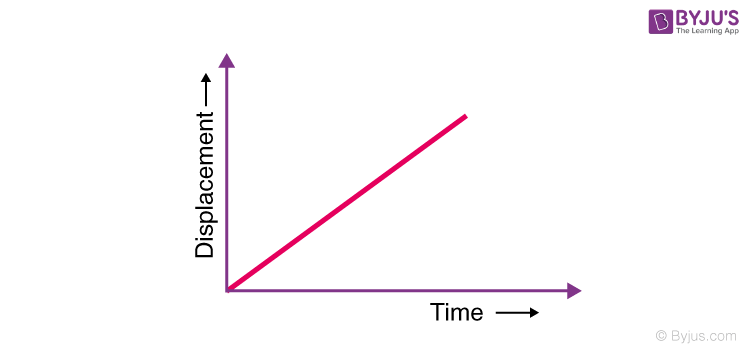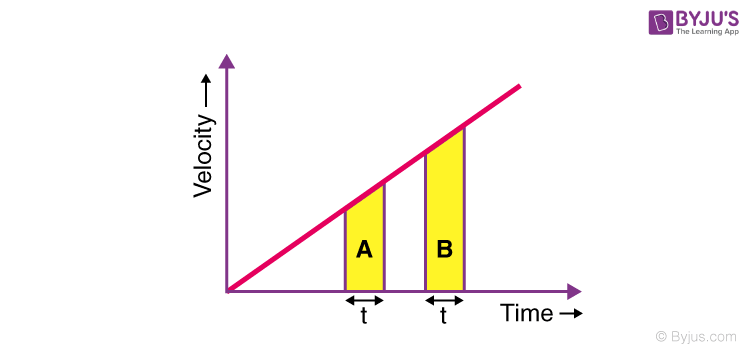# Comparison Between Uniform And Non-Uniform Motion

If we analyze our motion from home to office we can visualize that we are not moving with a constant velocity all the time. Sometimes we are moving at a higher velocity, while at other times like in crowded places like markets the velocity will be less. But we can always define an average velocity for the motion. Based on this we can classify the motion to be uniform or non-uniform. In this article, let us know more about uniform and non-uniform motion.

### Uniform Motion:

In a uniform motion, the body covers equal distances in equal intervals of time. That means it has a constant velocity over the given period of time.

Examples of Uniform Motion:

1. Movement of the ceiling fan’s blades.
2. The motion of the earth around the sun.
3. Pendulum with equivalent amplitude on either side.

By looking at the x – t, and v – t graph we can say what kind of motion it is. First, we will take x – t graph:From the graph, we can see that the slope is constant. Therefore,

$$\begin{array}{l}\frac{Change ~in ~displacement}{Change~ in ~time} = Constant\end{array}$$

So the motion is uniform. Similarly, if we take a v – t graph and the plot is horizontal or parallel to the time axis then the motion is uniform as it covers equal distance in equal interval of time.

### Non-Uniform Motion:

As the name suggests that a motion in which the body covers unequal distances in equal intervals of time is known as a non-uniform motion.

Examples of Non-Uniform Motion:

1. Asteroid movement.
2. Bouncing ball.
3. Running horse.

Let’s see the following v – t graph:We know are under v – t graph represents displacement of the body. As we can see for the same time interval the area under A and B are different. So this is a non-uniform motion.

### Average Velocity

We usually define average velocity with respect to non-uniform motion. The average velocity formula is:

$$\begin{array}{l}Average ~Velocity = \frac{Change~ in ~displacement}{Time~ Taken}\end{array}$$

From the equation of motion,

$$\begin{array}{l}Displacement = ut ~+~\frac{1}{2}at^2\end{array}$$

$$\begin{array}{l}Average~ Velocity = \frac{ut ~+ ~\frac{1}{2} at^2}{t}\end{array}$$

$$\begin{array}{l}=u ~+~\frac{at}{2}\end{array}$$

From first equation of motion,

$$\begin{array}{l}Average~ velocity = u ~+~ \frac{v ~-~ u}{2}\end{array}$$

$$\begin{array}{l}Average~ Velocity = \frac{v~+~ u}{2}\end{array}$$

Where, v = Final Velocity

u = Initial Velocity

Similarly, we can define average speed. The formula for average speed is given us:

$$\begin{array}{l}Average~ speed = \frac{Change~ in~ distance}{Time~ Taken}\end{array}$$

## See the video below, to understand uniform and non-uniform motion## Frequently Asked Questions – FAQs

Q1

### Define uniform motion?

If the body covers equal distances in equal intervals of time, it is known as uniform motion.
Q2

### Define non-uniform motion?

A motion in which the body covers unequal distances in equal intervals of time is known as a non-uniform motion.

Q3

### Give the formula to calculate the average speed.

$$\begin{array}{l}Average~ speed = \frac{Change~ in~ distance}{Time~ Taken}\end{array}$$
Q4

### By referring to x – t, and v – t graphs, can v predict the type of motion?

Yes, we can predict the type of motion seeing x – t, and v – t graphs.
Q5

Uniform motion.

## The complete quiz of the chapter Motion Class 9 Science## Top 10 NTSE Important Questions on Motion Class 9Hope you have understood uniform and non-uniform motion. To learn more about different types of equations, get connected to BYJU’S – The learning app.

Test your Knowledge on Uniform Circular Motion